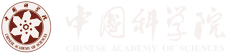Skip to content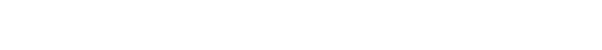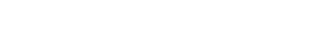Amplitude (Higgs) mode in a three-dimensional dimerized antiferromagnet

Date：05-04-2017 Print

The discovery of the Higgs boson of the Standard Model is arguably the greatest event in physics yet this century. In condensed matter, we have had Higgs'' modes for several decades, in the form of amplitude excitations in superconductors, charge-density-wave materials and magnets. However, it is only within the last decade that we have developed the ability to control the Higgs mode, by driving systems through their quantum phase transition and simultaneously probing their excitation spectrum. These experiments have now been achieved in atomic condensates and superconductors in two dimensions and quantum antiferromagnets in three, although their interpretation remains far from unambiguous.

The Higgs mode is one of the universal consequences of the spontaneous breaking of a continuous symmetry, which occurs at a quantum critical point (QCP). Experimental studies of quantum critical (QC) phenomena are difficult, due to the need for fine control over both the temperature and a quantum tuning parameter in the region of the QCP. Studies of the Higgs mode are inordinately difficult, first because they are dynamical and second because the massive Higgs mode has a tendency to decay into the massless Goldstone modes that are also present in the symmetry-broken phase, and this explains the ambiguities mentioned above.

Nonetheless, recent experimental studies of the pressure-induced magnetic QCP in three-dimensional dimerized antiferromagnets mandate a theoretical response. This situation is at the upper critical dimension of the system, which means two things. The first is that the Higgs mode decays only marginally'' into Goldstone bosons; it can therefore be studied, but its width scales with its energy and thus its spectral function is intrinsically complex. Second, the QC phenomena are subject to logarithmic corrections, whose quantitative study is an extremely difficult task due to their weak functional forms and strong sensitivity to error bars and exact extrapolations.

Recently, a research term (referred as “we” below ) comprised of Yan Qi Qin, Zi Yang Meng (IOP, CAS), Bruce Normand (Renmin University and PSI) and Anders W. Sandvik (Boston University), investigated the problem with large scale quantum Monte Carlo simulations. From a numerical standpoint, we have risen to the second challenge by performing large-scale quantum Monte Carlo simulations with state-of-the-art supercomputing facilities (TianHe1A platform at National supercomputer center at Tianjin) and careful finite-size extrapolations, to approach points unprecedentedly close to the QCP. Concerning the first challenge, the extraction of spectral information has always been a weak point of Monte Carlo studies, requiring as it does the solution of an ill-defined inversion problem, i.e., analytic continuation from imaginary time to real frequency.

While progress has been made over the decades, complex spectral functions have remained beyond the boundaries of what can be achieved with quantitative accuracy. Here we have implemented a new stochastic analytic continuation approach which crosses this boundary for the system at hand, and thus constitutes the first test of this method on a cutting-edge theoretical problem.

The results of this numerical effort are clear: our high-precision spectral data allow us to provide an unbiased demonstration that (i) the Higgs mode energy obeys a universal ratio to the triplon gap, (ii) that it exhibits logarithmic scaling corrections, (iii) that its intrinsic width is precisely critically damped, and (iv) that its spectral weight has a characteristic low-frequency scaling, all of which serve to verify the predictions of perturbative renormalization-group field-theoretical calculations.

To summarize, the universal behavior we observe is of interest to a broad cross-section of the community, spanning theory, numerics, and experiment in hard and soft condensed matter, cold atoms, field theory, and high-energy physics. The nonuniversal constants we deduce are of particular interest for experimental realization. The numerical advances we introduce lie beyond the limits of what was previously possible and open new horizons in the quantitative study of quantum critical dynamics.

This work has been accepted to be published in Phys. Rev. Lett., and will be published soon.

This study is supported by the Ministry of Science and Technology of China under Grant No. 2016YFA0300502, the National Science Foundation of China under Grant Nos. 11421092 and 11574359, the Condensed Matter Theory Visitors Program of Boston University, and the NSF under Grant No. DMR-1410126. Numerical calculations were performed on the Tianhe-1A platform at the National Supercomputer Center in Tianjin.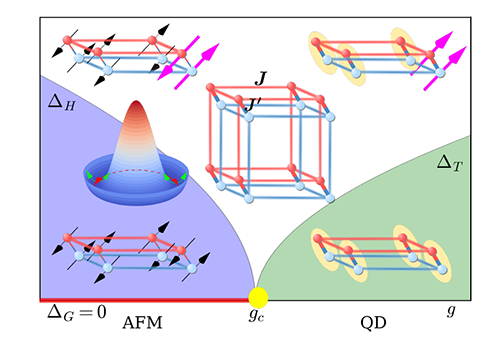Fig.1 Schematic representation of excitation processes and corresponding gaps in a dimerized antiferromagnet. The ratio g = J'/J of the intra- and inter-dimer coupling constants controls a QPT from an AFM to a QD state. In the AFM phase, the excitations are two gapless spin waves (Goldstone modes, Delta_G = 0) plus an amplitude mode at energy Delta_H, which correspond respectively to axial and radial fluctuations in the Mexican hat'' potential. In the QD phase, singlet--triplet dimer excitations have a gap Delta_T.Fig2： Triplon gaps in the thermodynamic limit (blue triangles), shown vs |g – g_c|/g_c. The red line shows a pure mean-field (square-root) form, the blue line includes the log correction with fitted exponent hat{\nu} = 0.230(2), and green points show the extrapolated Higgs energy, Delta_H, obtained for values of g < g_c (AFM phase) mirroring those g>g_c (QD phase). The blue dashed line is the log-corrected Delta_T result multiplied by sqrt{2}.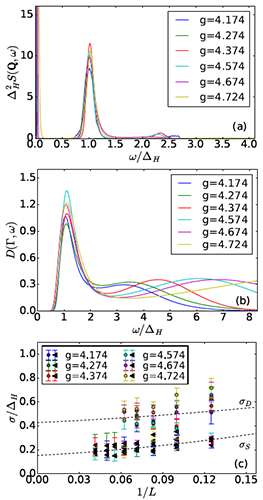Fig. 3: (a) The scaled spectrum Delta_H^2 S(Q,omega/Delta_H) calculated with L = 24 for a range of g values. (b) D(Gamma,omega/Delta_H) calculated with L = 16. (c) Scaled peak widths shown vs 1/L. Circles and triangles are obtained from D(Gamma,omega/Delta_H) and S(Q,omega/Delta_H), respectively. The dashed curves are second-order polynomial fits to error-weighted average widths.

Contact:
Institute of Physics
ZI YANG MENG
Email：zymeng@iphy.ac.cn
Key word:
Higgs amplitude mode, quantum phase transition, quantum Monte Carlo, analytic continuation

Abstract:
The amplitude (Higgs) mode is a ubiquitous collective excitation related to spontaneous breaking of a continuous symmetry. We combine quantum Monte Carlo (QMC) simulations with stochastic analytic continuation to investigate the dynamics of the amplitude mode in a three-dimensional dimerized quantum spin system. We characterize this mode by calculating the spin and dimer spectral functions near the quantum critical point, finding that both the energies and the intrinsic widths satisfy field-theoretical scaling predictions. While the line width of the spin response is close to that observed in neutron scattering experiments on TlCuCl_3, the dimer response is significantly broader. Our results demonstrate that highly non-trivial dynamical properties are accessible by modern QMC and analytic continuation methods.